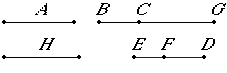# Proposition 89

To find the fifth apotome.

Set out a rational straight line A, and let CG be commensurable in length with A. Then CG is rational.

Set out two numbers DF and FE such that DE again has to neither of the numbers DF nor FE the ratio which a square number has to a square number, and let it be contrived that FE is to ED as the square on CG is to the square on GB.X.6

Then the square on GB is also rational. Therefore BG is also rational.

X.9

Now since DE is to EF as the square on BG is to the square on GC, while DE does not have to EF the ratio which a square number has to a square number, therefore neither does the square on BG have to the square on GC the ratio which a square number has to a square number. Therefore BG is incommensurable in length with GC.

X.73

And both are rational, therefore BG and GC are rational straight lines commensurable in square only. Therefore BC is an apotome.

I say next that it is also a fifth apotome.

Let the square on H be that by which the square on BG is greater than the square on GC.

V.19,Cor.

Since the square on BG is to the square on GC as DE is to EF, therefore, in conversion, ED is to DF as the square on BG is to the square on H.

X.9

But ED does not have to DF the ratio which a square number has to a square number, therefore neither has the square on BG to the square on H the ratio which a square number has to a square number. Therefore BG is incommensurable in length with H.

And the square on BG is greater than the square on GC by the square on H, therefore the square on GB is greater than the square on GC by the square on a straight line incommensurable in length with GB.

X.Def.III.5

And the annex CG is commensurable in length with the rational straight line A set out, therefore BC is a fifth apotome.

Therefore the fifth apotome BC has been found.

Q.E.F.

## Guide

This proposition is not used in the rest of the Elements.